• 文章目录前言哈夫曼树(最优二叉树)定义与原理树的路径长度带权路径长度构造哈夫曼树哈夫曼树生成代码 前言 二叉树是树结构中的一种特殊形式，适用于折半查找、真假、对错等具有两种情况的事物进行建模。 比如需要对...


文章目录
前言哈夫曼树(最优二叉树)定义与原理树的路径长度带权路径长度
构造哈夫曼树
哈夫曼树生成代码

前言
二叉树是树结构中的一种特殊形式，适用于折半查找、真假、对错等具有两种情况的事物进行建模。 比如需要对学生考试得分评不及格、及格、中等、良好、优秀这几个模糊分数的评级，我们可以很快用如下的代码实现：
if score < 60:
print("不及格")
elif score < 70:
print("及格")
elif score < 80:
print("中等")
elif score < 90:
print("良好")
else:
print("优秀")

我们用二叉树可以表示如下：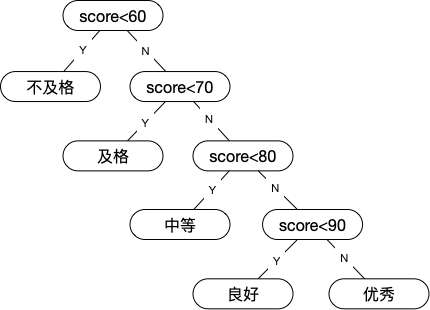我们上面建模出来的二叉树在查找判断中效率是否是最优的呢？ 我们结合现实场景进行思考，一场考试下来，发现分数占比如下：
分数0~5960~6970~7980~8990~100所占比例5%15%40%30%10%
采用上面的二叉树进行判断70分以上的分数，有80%至少要进过3次以上的判断才能得到结果。 那么有没有更好方式进行查找判断呢？我们可以调整判断顺序，将占比多的70~79的判断提前，然后再判断80 ~ 89，这样按照占比从大到小一次判断。按照这种方式我们得到如下的二叉树：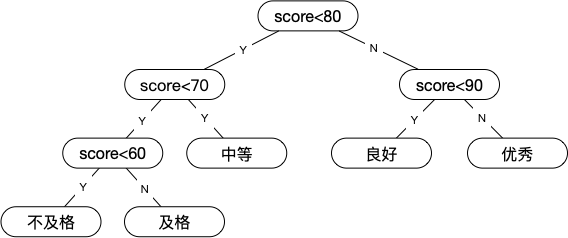我们优化过后的二叉树相比第一个二叉树效率高了很多。 那么如何构建一个最优二叉树呢？这就是接下来要介绍的内容，哈夫曼树。
哈夫曼树(最优二叉树)
哈夫曼树就是我们平时说的最优二叉树。 哈夫曼(David Huffman)是美国数学家，在1952年发明了哈夫曼编码，而哈夫曼编码就是基于哈夫曼树得来的，本文只介绍哈夫曼树。
定义与原理
树的路径长度
从树中一个节点到另一个节点之间的分支构成两个节点之间的路径，路径上的分支数目称为路径长度。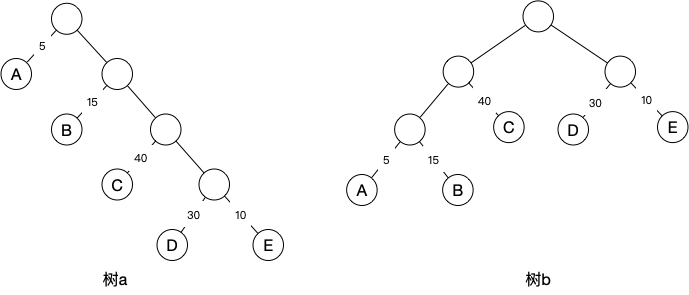树a的根节点到D节点，路径长度为4。树b的根节点到节点D长度为2。树的路径长度为根节点到各节点的路径长度之和。 树a的树路径长度为1+1+2+2+3+3+4+4=20。树b的树路径长度为1+2+3+3+2+1+2+2=16。
带权路径长度
树的带权路径长度记为WPL(Weighted Path Length of Tree) 节点的带权路径长度为根节点到该节点路径长度与该节点的权的乘积。树的带权路径长度为各叶子节点的带权路径长度之和。 树a的WPL=1x5+2x15+3x40+4x30+4x10=315 树b的WPL=3x5+3x15+2x40+2x30+2x10=220 树的WPL越小，那么树就越优。
构造哈夫曼树
假定我们按照A5、B15、C40、D30、E10生成一棵哈夫曼树。 按照如下步骤操作：
将所有权重节点按照权重由小到大进行排序，即：A5,E10,B15,D30,C40将最左的两个节点按照左小右大作为新节点N1的左右两个子节点。N1的权重=5+10=15。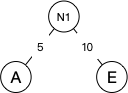将N1替换序列的A和E并加入。重复2步骤，将N1和B作为新节点N2的两个子节点。N2的权重=15+15=30。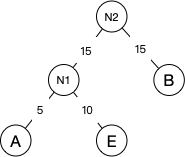然后继续重复2步骤，将N2替换N1和B加入到序列中，并将N2与D作为新节点N3的两个子节点。N3的权重=30+30=60。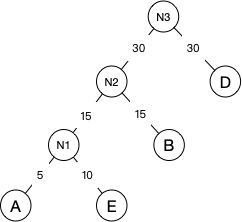然后继续重复2步骤，将N3替换N2和D并加入到序列。将N3和E作为新节点R的两个子节点。因为N3的权重为60，C的权重为40，所以C作为R的左子节点，N3作为右子节点。并且R已经是根节点了，所以最终的哈夫曼树就如下图：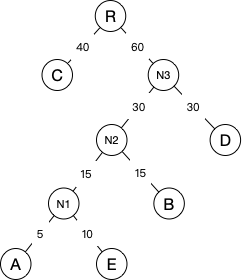该树的WPL=1x40+2x30+3x15+4x10+4x5=205 比前面的树b的WPL=225还要少15，所以该树就是最优的哈夫曼树了。
我们对创建哈夫曼树步骤总结如下：
将给定的n个权值构成n棵只有一个节点的树，并根据权值由小到大进行排序。取最左遍权值最小的两棵树作为左右子树构成一颗新二叉树，新二叉树的权值为两棵字数的权值和。将2步骤构造的新树的两个子树删除，将构造的新树放入序列的最左边。重复2、3步骤，直到所有树合并为一棵树为止。最终的树就是哈夫曼树，也就是最优二叉树。
哈夫曼树生成代码
代码用Python3实现如下：
import functools

class TreeNode:

def __init__(self, data, weight) -> None:
self.data = data  # 数据
self.weight = weight  # type: int #权重
self.left = None  # 左子节点
self.right = None  # 右子节点

def __str__(self) -> str:
return self.data

def cmp(a, b):
"""
排序
"""
if a.weight > b.weight:
return 1
elif a.weight < b.weight:
return -1
else:
return 0

def gen_huffman_tree(_trees, depth=0):
"""
构建哈夫曼树
:param depth: 深度
:param _trees: 树集
"""
if depth == 0:
print('对' + ','.join([str(item) for item in tree]) + '树集生成哈夫曼树。数据|权重')
depth = depth + 1  # 深度+1
if len(_trees) == 1:
return _trees
_trees = sorted(_trees, key=functools.cmp_to_key(cmp))
left_sub = _trees
right_sub = _trees
new_node_weight = left_sub.weight + right_sub.weight  # 新树权重
# 构建新树
new_node = TreeNode('N%s|%s' % (str(depth), str(new_node_weight)), new_node_weight)
new_node.left = left_sub
new_node.right = right_sub
# 删除最左两个树
_trees.remove(left_sub)
_trees.remove(right_sub)
# 新树插入到最左序列
_trees.insert(0, new_node)
# 递归构建下一个树，直到只剩下一棵树
return gen_huffman_tree(_trees, depth)

def layer_order_traverse(_layer_nodes):
"""
按层遍历
:param _layer_nodes: 当前层节点集合
:type _layer_nodes: list
"""
if _layer_nodes is None or len(_layer_nodes) == 0:
return
_childs = []  # 子集
for _node in _layer_nodes:  # 遍历传入的当前层所有节点
print(_node.data, end=',')
if _node.left:
_childs.append(_node.left)
if _node.right:
_childs.append(_node.right)
layer_order_traverse(_childs)

if __name__ == '__main__':
tree = [
TreeNode('A|5', 5),
TreeNode('B|15', 15),
TreeNode('C|40', 40),
TreeNode('D|30', 30),
TreeNode('E|10', 10)
]
huffman_tree = gen_huffman_tree(tree)
print('按层遍历哈夫曼树：', end='')
layer_order_traverse([huffman_tree])
print('\b' * 1, end='')


该代码将上面的例子A5、B15、C40、D30、E10树集生成了如下最优二叉树。代码允许后的结果如下：
对A|5,B|15,C|40,D|30,E|10树集生成哈夫曼树。数据|权重
按层遍历哈夫曼树：N4|100,C|40,N3|60,N2|30,D|30,N1|15,B|15,A|5,E|10

生成了哈夫曼树之后，按层遍历打印出了树的每个节点，第一个N4为根节点，可以看出和上面的二叉树的顺序是一一对应的。 按层遍历算法可以看下二叉树遍历算法。
展开全文赫夫曼树
• （注意：“带权路径长度最短”是在度相同的树中比较所得，因此有“最优二叉树”、“最优三叉树”等之称）满二叉树不一定是哈夫曼树。 特点： 哈夫曼树中权值越大的叶子离根越近 哈夫曼树不唯一 哈夫曼树中只有度为0...


文章目录

哈夫曼树的一些基本概念
哈夫曼树的构造（哈夫曼算法）
哈夫曼树的存储及创建
哈夫曼树的应用——哈夫曼编码

不同的判别树的效率是不同的。哈夫曼树的一些基本概念展开全文数据结构
• 哈夫曼编码哈夫曼树（最优二叉树）哈夫曼编码（前缀编码） 哈夫曼树（最优二叉树） 具有最小带权路径长度的二叉树称为哈夫曼树 权值越大的叶子节点越靠近根节点 权值越小的叶子节点越远离根节点 总是将最小...


哈夫曼树&哈夫曼编码
哈夫曼树（最优二叉树）哈夫曼编码（前缀编码）

哈夫曼树（最优二叉树）
具有最小带权路径长度的二叉树称为哈夫曼树  权值越大的叶子节点越靠近根节点  权值越小的叶子节点越远离根节点  总是将最小权值和次最小权值的两个节点合并，依次往上构造树，这样根节点的权值就是最小权值  假如哈夫曼树有n0个叶子节点，那么这棵树的总结点个数n=2*n0-1
typedef struct{
int weight;
int parent;
int lchild;
int rchild;
}HNode;

void Create_HuffMTree(HNode HFMTree[],int n){       //n为叶子结点个数
int x1,x2;  //x1和x2存储最小和次最小权值
int m1,m2;  //m1和m2存储位置
int i,j;
for(i=0;i<2*n-1;++i){       //HFMTree 初始化
HFMTree[i].weight=0;
HFMTree[i].lchild=-1;
HFMTree[i].rchild=-1;
HFMTree[i].parent=-1;
}
for(i=0;i<n;++i)
cin>>HFMTree[i].weight; //输入n个叶子节点的权值
for(i=0;i<n-1;++i){
x1=x2=INT_MAX;
m1=m2=0;
for(j=0;j<n+i;++j){
if(HFMTree[i].parent==-1&&HFMTree[j].weight<x1){
x2=x1;
m2=m1;
x1=HFMTree[j].weight;
m1=j;
}
else if(HFMTree[i].parent==-1&&HFMTree[j].weight<x2){
x2=HFMTree[j].weight;
m2=j;
}
}
HFMTree[m1].parent=n+i;HFMTree[m2].parent=n+i;          //合并
HFMTree[n+i].weight=HFMTree[m1].weight+HFMTree[m2].weight;
HFMTree[n+i].lchild=m1;HFMTree[n+i].rchild=m2;
}
}


哈夫曼编码（前缀编码）
规定哈夫曼树中的左分支代表0，右分支代表1，从根节点到每个叶子节点所经过的路径分支组成的0和1的序列便为该节点对应字符的编码
const int n=10;         //叶子节点个数

typedef struct{
int bit[n];
int start;
}HCode;

HNode HFMTree;          //设已经建立好的哈夫曼树已经存在HFMTree中

void HuffmanCode(HNode HFMTree[],HCode HuffCode[]){
HCode cd;
int i,j,c,p;
for(i=0;i<n;++i){
cd.start=n-1;
c=i;
p=HFMTree[c].parent;
while(p!=-1){
if(HFMTree[p].lchild==c)
cd.bit[cd.start]=0;
else
cd.bit[cd.start]=1;
cd.start--;
c=p;
p=HFMTree[c].parent;
}
for(j=cd.start+1;j<n;++j)
HuffCode[i].bit[j]=cd.bit[j];
HuffCode[i].start=cd.start+1;
}
}

展开全文数据结构 c++ 算法
• 哈夫曼树：给定一组具有确定权值的叶子结点，带权路径长度最小的二叉树哈夫曼树的特点： 1 权值越大的叶子结点越靠近根结点，而权值越小的叶子结点越远离根结点。 2 只有度为0（叶子结点）和度为2（分支结点）...
最优二叉树： 叶子结点的权值：对叶子结点赋予的一个有意义的数值量。 二叉树的带权路径长度：设二叉树具有n个带权值的叶子结点，从根结点到各个叶子结点的路径长度与相应叶子结点权值的乘积之和。 哈夫曼树：给定一组具有确定权值的叶子结点，带权路径长度最小的二叉树。 哈夫曼树的特点： 1 权值越大的叶子结点越靠近根结点，而权值越小的叶子结点越远离根结点。 2 只有度为0（叶子结点）和度为2（分支结点）的结点，不存在度为1的结点. 哈夫曼算法基本思想： ⑴ 初始化：由给定的n个权值{w1，w2，…，wn}构造n棵只有一个根结点的二叉树，从而得到一个二叉树集合F＝{T1，T2，…，Tn}； ⑵ 选取与合并：在F中选取根结点的权值最小的两棵二叉树分别作为左、右子树构造一棵新的二叉树，这棵新二叉树的根结点的权值为其左、右子树根结点的权值之和； ⑶ 删除与加入：在F中删除作为左、右子树的两棵二叉树，并将新建立的二叉树加入到F中； ⑷ 重复⑵、⑶两步，当集合F中只剩下一棵二叉树时，这棵二叉树便是哈夫曼树。 设置一个数组huffTree[2n-1]保存哈夫曼树中各点的信息，数组元素的结点结构 weight：权值域，保存该结点的权值； lchild：指针域，结点的左孩子结点在数组中的下标； rchild：指针域，结点的右孩子结点在数组中的下标； parent：指针域，该结点的双亲结点在数组中的下标； struct element { int weight; int lchild, rchild, parent; }; 1：数组huffTree初始化，所有元素结点的双亲、左 右孩子都置为-1； 2. 数组huffTree的前n个元素的权值置给定值w[n]； 3. 进行n-1次合并 3.1 在二叉树集合中选取两个权值最小的根结点， 其下标分别为i1, i2； 3.2 将二叉树i1、i2合并为一棵新的二叉树k(初值为n；依次递增)； 代码：
void HuffmanTree(element huffTree[ ], int w[ ], int n ) {
for (i=0; i<2*n-1; i++) {
huffTree [i].parent= -1;
huffTree [i].lchild= -1;
huffTree [i].rchild= -1;
}
for (i=0; i<n; i++)
huffTree [i].weight=w[i];
for (k=n; k<2*n-1; k++) {
Select(huffTree, &i1, &i2); //选出两个最小的权值。
huffTree[k].weight=huffTree[i1].weight+huffTree[i2].weight;
huffTree[i1].parent=k;
huffTree[i2].parent=k;
huffTree[k].lchild=i1;
huffTree[k].rchild=i2;
}
}

哈夫曼编码： 编码：给每一个对象标记一个二进制位串来表示一组对象。 例：ASCII，指令系统 等长编码：表示一组对象的二进制位串的长度相等。 不等长编码：表示一组对象的二进制位串的长度不相等。 前缀编码：一组编码中任一编码都不是其它任何一个编码的前缀 。 前缀编码保证了在解码时不会有多种可能。 线索二叉树： 在有n个结点的二叉链表中共有2n个链域，但只有n-1个有用的非空链域，其余n+1个链域是空的。 **我们可以利用剩下的n+1个空链域来存放遍历过程中结点的前驱和后继信息。 线索：将二叉链表中的空指针域指向前驱结点和后继结点的指针被称为线索； 线索化：使二叉链表中结点的空链域存放其前驱或后继信息的过程称为线索化； 线索二叉树：加上线索的二叉树称为线索二叉树 itag=0: lchild指向该结点的左孩子 itag=1: lchild指向该结点的前驱结点。 rtag=0: rchild指向该结点的右孩子 rtag=1: rchild指向该结点的后继结点
enum flag {Child, Thread}; //枚举类型：枚举常量Child=0,Thread=1; template  struct ThrNode { T data; ThrNode *lchild, *rchild; flag ltag, rtag; }; 中序线索链表： 建有带有标志位的二叉树：
template <class T>ThrNode<T>* InThrBiTree<T>::Creat( ){
ThrNode<T> *root;
T ch;
cout<<"请输入创建一棵二叉树的结点数据"<<endl;
cin>>ch;
if (ch=="#") root = NULL;
else{
root=new ThrNode<T>;
root->data = ch;
root->ltag = Child; root->rtag = Child;
root->lchild = Creat( );
root->rchild = Creat( );
}
return root;
}

函数设置形参root和全局变量pre，分别表示要处理的树的根结点和其前驱结点 如果root!=NULL 中序线索化root的左子树 中序线索化root本身 如果root->lchild= =NULL root->left=pre,root->ltag=1; 如果root->rchild==NULL,root->rtag=1, 如果pre！=NULL， 并且pre->rtag=1， pre->rchild=root,; pre=root 中序线索化root的右子树
中序线索化二叉树：递归
template <class T>  void ThrBiTree<T>::ThrBiTree (ThrNode<T>*root) {
if (root==NULL) return;         //递归结束条件

ThrBiTree(root->lchild);
if (!root->lchild){             //对root的左指针进行处理
root->lchild = pre;        //设置pre的前驱线索
}
if(pre != NULL){
}
pre = root;
ThrBiTree(root->rchild);
}

template <class T>
InThrBiTree<T>::InThrBiTree( )
{
//ThrNode<T>* pre = NULL;
this->root = Creat( );
ThrBiTree(root);
}

在中序线索二叉树中查找结点的中序遍历的后继：
template <class T> ThrNode<T>* InThrBiTree<T>::Next(ThrNode<T>* p)
{
ThrNode<T>* q;  //要查找的p的后继
else{
q = p->rchild;
while (q->ltag==Child)	{
q = q->lchild;
}
}
return q;
}

中序遍历中序线索二叉树

template <class T>
void InThrBiTree<T>::InOrder(ThrNode<T> *root){
ThrNode<T>* p = root;
if (root==NULL)  return;
while (p->ltag==Child)   {       p = p->lchild;    }
cout<<p->data<<" ";
while (p->rchild!=NULL) {
p = Next(p);
cout<<p->data<<" ";
}
cout<<endl;
}

展开全文• 哈夫曼树一、哈夫曼树基本概念二、哈夫曼树的构造算法 一、哈夫曼树基本概念 （1）路径：从树中的一个结点到另一个结点之间的分支构成这两个结点之间的路径 （2）路径长度：路径上的分支数目称作路径长度。 （3...数据结构 算法
• 给定n个带权值的叶子节点，构造称一棵二叉树，带权路径长度达到最小的二叉树，称为最优二叉树，也称为哈夫曼树。 构造方法： 将这n个叶子节点看作n个森林 在森林中选出两个权值最小的节点作为左右子树合并成一个...
• 哈夫曼树 ...哈夫曼树又称最优二叉树，是一种带权路径长度最短的二叉树。所谓树的带权路径长度，就是树中所有的叶结点的权值乘上其到根结点的路径长度（若根结点为0层，叶结点到根结点的路径长度为叶哈夫曼 哈夫曼算法
• 哈夫曼树：给定一组具有确定权值的叶子结点，带权路径长度最小的二叉树哈夫曼树的特点： 权值越大的叶子结点越靠近根结点，而权值越小的叶子结点越远离根结点。 只有度为0（叶子结点）和度为2（分支结点）的结点...
• 哈夫曼树（最优二叉树）】 --- 概念以及构造哈夫曼树产生的背景准备概念哈夫曼二叉树的概念以及构造 哈夫曼树产生的背景 准备概念 哈夫曼二叉树的概念以及构造 ...
• 哈夫曼树又被称为最优二叉树，是一类带权路径最短的二叉树哈夫曼树二叉树的一种应用，在信息检索中很常用。 相关概念 节点之间的路径长度：从一个节点到另一个节点之间的分支数量称为两个节点之间的路径长度...数据结构 java 算法
• 树与二叉树的应用：哈夫曼树带权路径长度：树的带权路径长度：哈夫曼树的定义：哈夫曼树的构造方法：哈夫曼树的性质：哈夫曼编码： 带权路径长度： 树的带权路径长度： 哈夫曼树的定义： 哈夫曼树的构造方法： ...
• 这时可使用哈夫曼树(最优二叉树)求解。 最优多叉树： 一般的哈夫曼树为二进制，当需要k进制时，每次选取最小的k个节点合并为一个节点即可，但此时可能出现最顶层反而没有填充满(不是最优解)的情况。可用添加 k-1-(n-...算法
• 哈夫曼树（最优二叉树） */ #include <iostream> #include <string> using namespace std; struct Node { int weight; int parent,lchild,rchild; }; class HuffmanTree { private: Node *hufftree...数据结构
•数据结构
• 哈夫曼树/最优二叉树（C语言实现） 带长路径长度（WPL）：设二叉树有n个叶子结点，每个叶子结点带有权值Wκ，从根结点到每个叶子结点的长度为lκ，则每个叶子结点的带权路径长度之和就是WPL=∑Wκ•lκ(n,i=1)。 ...
• Huffman tree(赫夫曼树、霍夫曼树、哈夫曼树、最优二叉树)flyfish 2015-8-1Huffman tree因为翻译不同所以有其他的名字 赫夫曼树、霍夫曼树、哈夫曼树 定义引用自严蔚敏《数据结构》 路径 从树中一个结点到另一个...Huffman 赫夫曼树 霍夫曼树
• 今晚也是帮她写好西邮导航睡不着，那就敲了一下哈夫曼树转化成二叉树的代码，其实理解了真的不难，我定义F为一个二级指针，用它指向结点的地址，创建很简单，输入数据data和权值weight，再把它的左右置为NULL；...
• 1，概念哈夫曼树，英文名 Huffman Tree, 又称最优二叉树（程序运行效率最优解）。2，相关术语路径： 树中一个结点到另一个结点之间的分支构成这两个结点之间的路径。 路径长度：路径上的分枝数目称作路径长度。 树...
• 树的带权路径长度：树中所有叶子结点的带权路径长度之和哈夫曼树的构造过程 （1）构造n棵只有根结点的二叉树，这n棵二叉树构成一个森林F （2）在F中选两棵根结点的权值最小的树作为左右子树构造一棵新的二叉树，且...算法 哈夫曼编码...